# Sector Of A Circle

Here we will learn about sectors of a circle, including how to find the area of a sector, the perimeter of a sector and solve problems involving sectors of circles.

There are also sector of a circle worksheets based on Edexcel, AQA and OCR exam questions, along with further guidance on where to go next if you’re still stuck.

## What is a sector of a circle?

A sector of a circle is formed when a circle is divided using two radii.

Each sector has an angle between the two radii. The sector with an angle less than 180 degrees is called a minor sector and the sector with an angle greater than 180 degrees is called a major sector. If the central angle formed equals 180 degrees, the two sectors would be semicircles.

For example,

In GCSE mathematics you will need to know how to solve problems involving the area of a sector and the perimeter of a sector. You may be asked to give exact answers in terms of \pi or using decimals.

### What is a sector of a circle?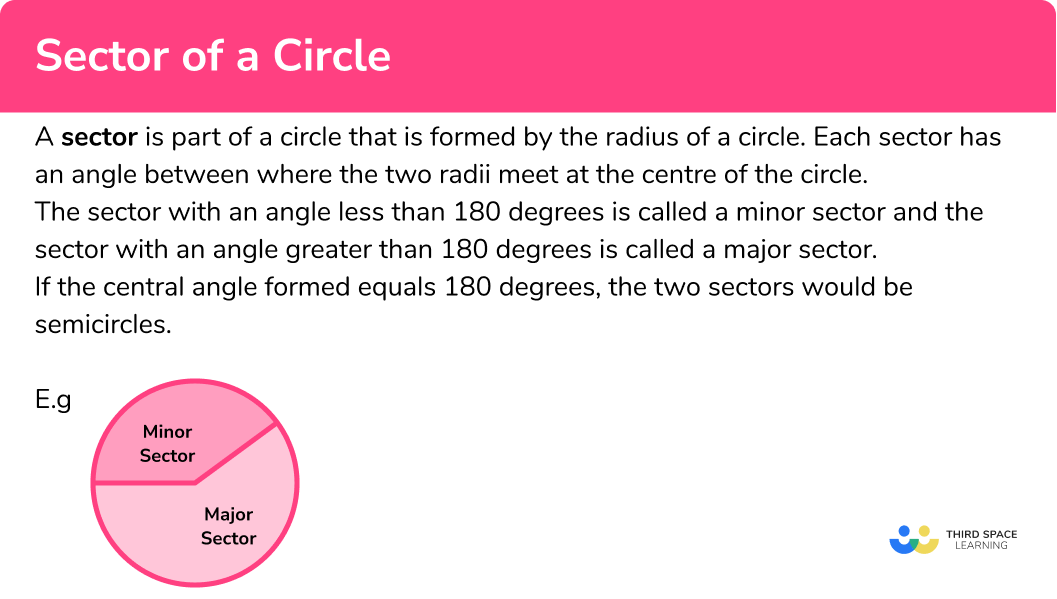## Area of a sector

The area of a sector is the space inside the section of the circle created by two radii and an arc. It is a fraction of the area of the entire circle.

The formula to find the area of a sector is,

\text{Area of a sector } = \frac{\theta}{360} \times \pi r^{2}.

\theta = \text{ angle of the sector}

r= \text{ radius of the circle}

## Perimeter of a sector

The perimeter of a sector is the distance around a sector.

We can calculate the perimeter of a sector by adding together the lengths of the two radii and the arc length of the sector.

To find the arc length of a sector we need to use the formula,

\text{Arc length } = \frac{\theta}{360} \times \pi\times d.

\theta = \text{ Angle of the sector}

d = \text{ Diameter of the circle}

Or

\text{ Arc length } = \frac{\theta}{360} \times 2\times\pi\times r.

\theta = \text{ Angle of the sector}

r = \text{ Radius of the circle}

The total perimeter of a sector formula would be,

\begin{aligned} \text{Perimeter of a sector } &= \text{ Arc length } + \text{ radius } + \text{ radius} \\\\ &= \frac{\theta}{360} \times \pi d+d \;\; \text{ or } \;\; \frac{\theta}{360} \times 2\pi r +2r \end{aligned}

## How to calculate the area of a sector of a circle

In order to calculate the area of a sector:

1. Find the length of the radius.
2. Find the size of the angle creating the sector.
3. Substitute the values you know into the formula for the area of a sector.

### Explain how to calculate the area of a sector of a circle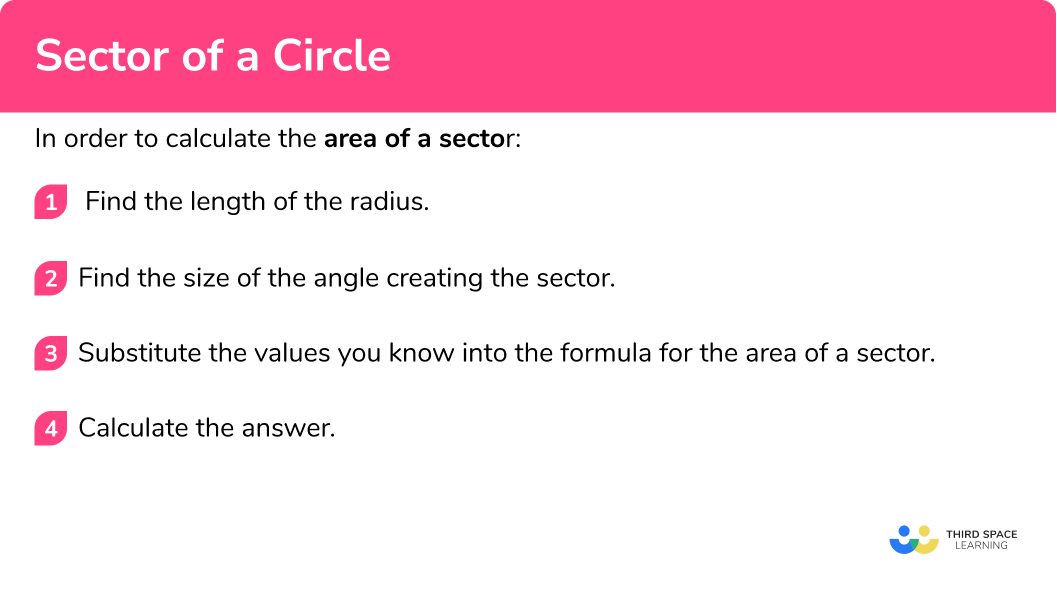Sector of a circle is part of our series of lessons to support revision on circles, sectors and arcs. You may find it helpful to start with the main circles, sectors and arcs lesson for a summary of what to expect, or use the step by step guides below for further detail on individual topics. Other lessons in this series include:

## Area of a sector of a circle examples

### Example 1: finding the area of a sector given the radius and angle

Calculate the area of the sector shown below.

1. Find the length of the radius.

2Find the size of the angle creating the sector.

Angle = 115^o

3Substitute the values you know into the formula for the area of a sector.

\begin{aligned} \text{Area of a sector } &=\frac{\theta}{360} \times \pi r^{2} \\\\ &= \frac{115}{360} \times \pi\times 8^{2} \\\\ &= \frac{184}{9}\pi \end{aligned}

\begin{aligned} \text{Area of a sector } &=\frac{184}{9}\pi \ cm^2 \\\\ &= 62.228...cm^2 \\\\ &=62.2 \ cm^2 \ (3 \ s.f) \end{aligned}

### Example 2: finding the area of a sector not given the angle

Calculate the area of the sector shown below.

The angle creating the sector is the angle included between the two radii.

To find it in this case we need to use the angle fact, angles around a point add to 360^{o}.

360-240=120

The angle creating the sector is 120^{o}.

\begin{aligned} \text { Area of a sector }&=\frac{\theta}{360} \times \pi \times r^{2} \\\\ &=\frac{120}{360} \times \pi \times 4^{2} \end{aligned}

\begin{aligned} &=16.75516083 \\\\ &=16.8 \ m^{2} \end{aligned}

### Example 3: finding the area of a sector not given the radius or angle

Below is a sector of a circle.

The diameter of the circle is 12 \ cm.

Calculate the area of the sector.

Diameter = 12 \ cm

The radius is half of the diameter, so the radius = 6 \ cm.

The angle creating the sector is the angle included between the two radii.

To find it in this case we need to use the angle fact, angles around a point add to 360^{o}.

360-205=155

The angle creating the sector is 155^{o}.

\begin{aligned} \text { Area of a sector }&=\frac{\theta}{360} \times \pi \times r^{2} \\\\ &=\frac{155}{360} \times \pi \times 6^{2} \end{aligned}

\begin{aligned} &=48.69468613 \\\\ &=48.7 \ cm^{2} \end{aligned}

## How to calculate the perimeter of a sector

In order to calculate the perimeter of a sector:

1. Find the length of the radius.
2. Find the size of the angle creating the sector.
3. Substitute the values you know into the formula for the arc length.

### Explain how to calculate the perimeter of a sector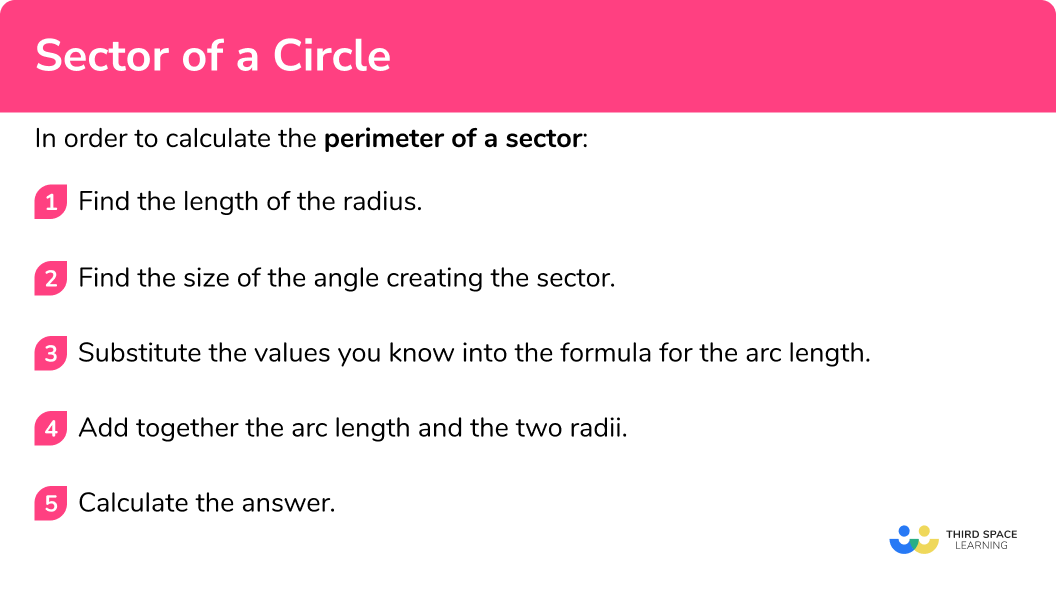## Perimeter of a sector examples

### Example 4: finding the perimeter of a sector given the angle

Calculate the perimeter of the sector shown below.

Angle = 117^o

\begin{aligned} \text{Arc length } &=\frac{\theta}{360} \times 2\times\pi \times r \\\\ &=\frac{117}{360} \times 2\times\pi \times 5.5 \\\\ &=\frac{143}{40} \pi \end{aligned}

Arc length: \frac{143}{40} \pi \ cm

\text{Total perimeter of sector } = \frac{143}{40} \pi + 5.5 +5.5 \ cm

\text{Total perimeter of sector } = \frac{143}{40} \pi + 11 \ cm

\begin{aligned} \text{Perimeter of a sector } &= \frac{143}{40} \pi + 11 \ cm \\\\ &=22.2311...cm \\\\ &=22.2 \ cm \end{aligned}

### Example 5: finding the perimeter of a sector not given the angle

Calculate the perimeter of the sector shown below.

The angle creating the sector is the angle included between the two radii.

To find it in this case we need to use the angle fact, angles around a point add to 360^{o}.

360-240=120

The angle creating the sector is 120^{o}.

\begin{aligned} \text{Arc length } &=\frac{\theta}{360} \times 2 \times \pi \times r \\\\ &=\frac{120}{360} \times 2 \times \pi \times 4 \\\\ &=8.37758041 \mathrm{~m} \end{aligned}

= 8.37758041+4+4

\begin{aligned} & =16.37758041 \\\\ & =16.4 \ m \end{aligned}

### Example 6: finding the perimeter of a sector not given the radius or angle

Below is a sector of a circle.

The diameter of the circle is 12 \ cm.

Calculate the perimeter of the sector.

Diameter = 12 \ cm

The radius is half of the diameter, so, the radius = 6cm.

Although we can use the radius or diameter as long as we use the correct formula for each.

The angle creating the sector is the angle included between the two radii.

To find it in this case we need to use the angle fact, angles around a point add to 360^{o}.

360-205=155

The angle creating the sector is 155^{o}.

\begin{aligned} \text{Arc length } &=\frac{\theta}{360} \times 2 \times \pi \times r \\\\ &=\frac{155}{360} \times 2 \times \pi \times 6 \\\\ &=16.23156204 \mathrm{~cm} \end{aligned}

= 16.23156204+6+6

\begin{aligned} &=28.23156204 \\\\ &=28.2 \ cm \end{aligned}

### Common misconceptions

• Confusing the segment/sector

A segment of a circle is made from a chord whilst a sector will have lines (radii) coming from the origin. Sectors are a portion of a circle – it can be helpful to think of a sector as a pizza slice.

• Finding the area of the whole circle not the area of the sector

Remember to find the fraction of the circle that makes the sector not just the area of the whole circle.

Use \ \frac{\theta}{360} \times \pi r^{2} \ not \ \pi r^2 \ to find the area of a sector.

• Finding the length of the arc not the perimeter of the sector

You must remember that the perimeter of the sector is the combined length of the arc and the two radii.

• Units

Remember the perimeter is a length and therefore the units will not be squared or cubed. Square units are for area and cubed units are for volume.

• Incorrect angle unit set on calculator

At GCSE all angles are measured in degrees. Make sure that your calculator has a small ‘d’ for degrees at the top of the screen rather than an ‘r’ for radians – these are not used until A Level.

### Practice sector of a circle questions

1. Find the area of the sector in terms of \pi.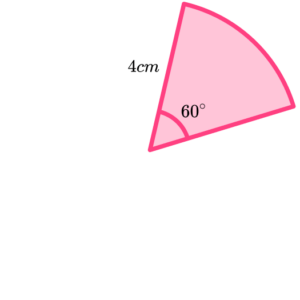16\pi \ cm^28\pi \ cm^2\frac{8}{3}\pi \ cm^28.4 \ cm^2Substitute the values we know into the formula for the area of a sector.

\begin{aligned} \text{ Area of a sector }&=\frac{\theta}{360} \times \pi \times r^{2} \\\\ &=\frac{60}{360} \times \pi \times 4^{2} \\\\ &=\frac{8}{3} \pi \ cm^{2} \end{aligned}

2. A sector has an area of 18\pi \ cm^2 and a radius of 12 \ cm. What is the angle of the sector?

45^{\circ}30^{\circ}60^{\circ}90^{\circ}Substituting the values we know into the formula for the area of a sector,

\frac{\theta}{360} \times \pi \times 12^{2}=18 \pi.

Solving for angle \theta ,

\theta=45^{\circ}.

3. A sector has an area of 21\pi \ cm^2 and an angle of 210^{\circ}. Find the radius of the sector.

12 \ cm6 \ cm9 \ cm36 \ cmSubstituting the values we know into the formula for the area of a sector,

\frac{210}{360} \times \pi \times r^{2}=21 \pi.

Solving for r ,

\begin{aligned} &r^{2}=36 \\\\ &r=\sqrt{36}=6 \mathrm{~cm}. \end{aligned}

4. Find the perimeter of the sector to 2 decimal places.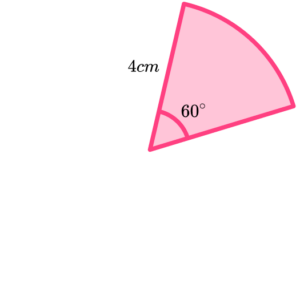8.19 \ cm16.38 \ cm10.09 \ cm12.19 \ cmSubstituting the values we know into the formula for the arc length,

\begin{aligned} \text{Arc length }&=\frac{\theta}{360} \times 2 \times \pi \times r \\\\ &=\frac{60}{360} \times 2 \times \pi \times 4 \\\\ &=4.188790205 \mathrm{~cm}. \end{aligned}

\begin{aligned} &=4.188790205+4+4 \\\\ &=12.188790205 \\\\ &=12.19 \mathrm{~cm}. \end{aligned}

5. A sector has a radius 5 \ cm and a perimeter 2\pi +10 \ cm. What was the angle of the sector?

72^{\circ}60^{\circ}90^{\circ}108^{\circ}Substituting the values we know into the formula for the total perimeter,

\left(\frac{\theta}{360} \times 2 \times \pi \times 5\right)+5+5=2 \pi+10.

Solving for \theta ,

\theta=72^{\circ}.

6. A sector has an angle 24^{\circ} and a perimeter 29.03 \ cm. What was the radius of the sector to the nearest centimetre?

24 \ cm12 \ cm9 \ cm10 \ cmSubstituting the values we know into the formula for the total perimeter,

\left(\frac{24}{360} \times 2 \times \pi \times r\right)+2 r=29.03.

Rearranging to change the subject of the formula to r,

\begin{aligned} &r\left(\frac{48 \pi}{360}+2\right)=29.03 \\\\ &r=29.03 \div\left(\frac{48 \pi}{360}+2\right) \\\\ &r=12.00142701 \\\\ &r=12 \mathrm{~cm}. \end{aligned}

### Sector of a circle GCSE questions

1. The diagram shows a sector with radius 5 \ cm and area 16 \ cm^{2}.

Find the perimeter of the sector, giving your answer to three significant figures.(4 marks)

Use of 16=\frac{\theta}{360} \times \pi \times 5^{2}.

(1)

Finding angle \theta =73.3385…

(1)

Use of \frac{\text{their angle}}{360} \times \pi \times 10 for arc length.

(1)

16.4 \ cm

(1)

2. The diagram shows triangle ABD and sector BDC.

Angle BDA = 50^{\circ}

Angle BDC = 30^{\circ}

AB = 7 \ cm.

Find the area of the sector BDC to three significant figures.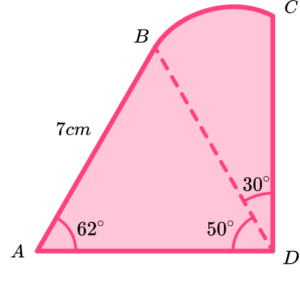(4 marks)

Use of sine rule \frac{BD}{\sin{62}}=\frac{7}{\sin{50}}.

(1)

BD = 8.068…

(1)

Use of \frac{30}{360} \times \pi \times "8.068"^{2}.

(1)

Area = 17.0 \ cm^2

(1)

3. A sector has a perimeter 50 \ cm and angle 200^{\circ}.

(3 marks)

Use of 50=\frac{200}{360} \times \pi \times d + d (or equivalent with r ).

(1)

Rearranging the formula to d=\frac{50}{\frac{200}{360} \times \pi +1} (or equivalent with r).

(1)

(1)

## Learning checklist

You have now learned how to:

• Calculate areas of a sector
• Calculate a property of a circle given the area of its sector
• Calculate the perimeter of a sector
• Calculate a property of a circle given the perimeter of a sector

## Still stuck?

Prepare your KS4 students for maths GCSEs success with Third Space Learning. Weekly online one to one GCSE maths revision lessons delivered by expert maths tutors.

Find out more about our GCSE maths tuition programme.